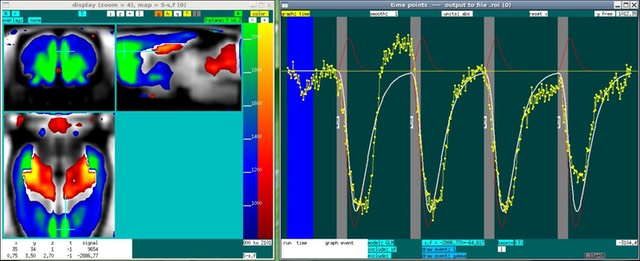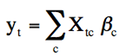## jip-glm

A time model for fMRI data using GLMjip-glm (a.k.a. glm) performs fMRI analysis using the general linear model (GLM):GLM is a standard way of solving equations that are linear in parameters ("Beta" above). The design matrix (X) contains the regressors, each of which can be any discrete function of time (t). For each regressor, there is a corresponding parameter, so temporal data (y) are described as a summation over regressors multiplied by coefficients (c). In other words, a data vector is described by summing a number of regressors, with a unique scale factor attached to each. GLM is simple, fast, and combines parameter estimation with statistical inference. Implementations, at least simple ones like this, are largely about book-keeping: the design matrix and the statistical tests should be constructed in a simple and intuitive way. Moreover, the GLM should be capable of reading small bytes of data to avoid memory limitations for large datasets, and also it should be integrated with a display package to view the analysis.

This GLM package was developed for a number of reasons, such as interactive cooperation with the display package for viewing pharmacological stimuli, and investigations of hemodynamic response functions using BOLD and IRON fMRI. These applications can be found in the literature under the names of the developer or colleague from the MGH Martinos Center who have published pre-clinical fMRI (or "phMRI").

For traditional ON/OFF stimuli, one generally would begin by creating the required set of files, running the GLM, and then displaying results as shown here.  For pharmacological stimuli, or for investigating connectivity using a seed region, one first might display the data in order to help define regressors interactively before creating the set of file required for program.

Joseph B. Mandeville, Athinoula A. Martinos Center for Biomedical Imaging at MGH/MIT/Harvard